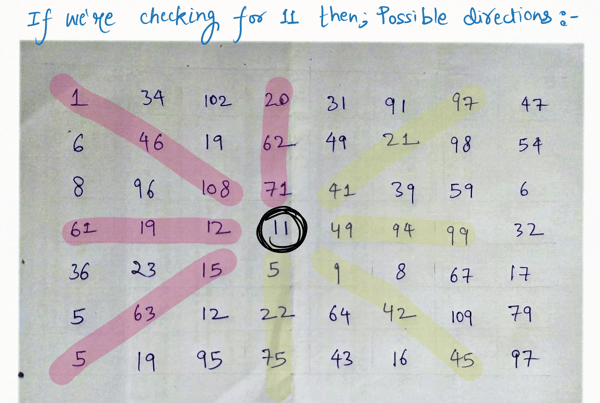Related Tags

project euler

# Project Euler 11: Largest product in a grid

Armaan Nougai

### Problem statement

Find the maximum product of four adjacent numbers in the same direction (horizontal, vertical, diagonal) from the grid given below.

GRID

### Question analysis

We have to optimally choose 4 adjacent numbers in the same direction. This could be:

• Vertical up
• Vertical down
• Horizontal forward
• Horizontal backward
• Ascending diagonal upper-part
• Ascending diagonal lower-part
• Descending diagonal upper-part
• Descending diagonal lower-part

### Solution

A simple approach with optimization can solve the given problem.

### Optimization

When checking for a number, we do not have to check all the possible directions. We only need to check the “yellow” directions:

• Vertical downward
• Horizontal forward
• Lower-part descending diagonal
• Upper-part ascending diagonal

This is because the “pink” quartet has either been checked before the number already or will be checked after the number.To run the code below, copy and paste the entire grid from the link at the top of this article into the input box.

"""
Coded by - Armaan Nougai
"""
# paste text file content in input for running this code

D_arr=[]
for j in range(20):
D_arr.append(list(map(int,input().split())))
if j!=19:input()

max_Product = 1
for row in range(20):
for column in range(20):
product = D_arr[row][column]

# horizontal forward
if column<17:
t_product = product*D_arr[row][column+1]*D_arr[row][column+2]*D_arr[row][column+3]
if max_Product<t_product:
max_Product=t_product

# vertical downward
if row<17:
t_product = product*D_arr[row+1][column]*D_arr[row+2][column]*D_arr[row+3][column]
if max_Product<t_product:
max_Product=t_product

# upper-part ascending diagonal
if row>2 and column<17:
t_product = product*D_arr[row-1][column+1]*D_arr[row-2][column+2]*D_arr[row-3][column+3]
if max_Product<t_product:
max_Product=t_product

# lower-part descending diagonal
if row<17 and column<17:
t_product = product*D_arr[row+1][column+1]*D_arr[row+2][column+2]*D_arr[row+3][column+3]
if max_Product<t_product:
max_Product=t_product

print(max_Product)            

Enter the input below

Python Implementation

RELATED TAGS

project euler

CONTRIBUTOR

Armaan Nougai
RELATED COURSES

View all Courses

Keep Exploring

Learn in-demand tech skills in half the time